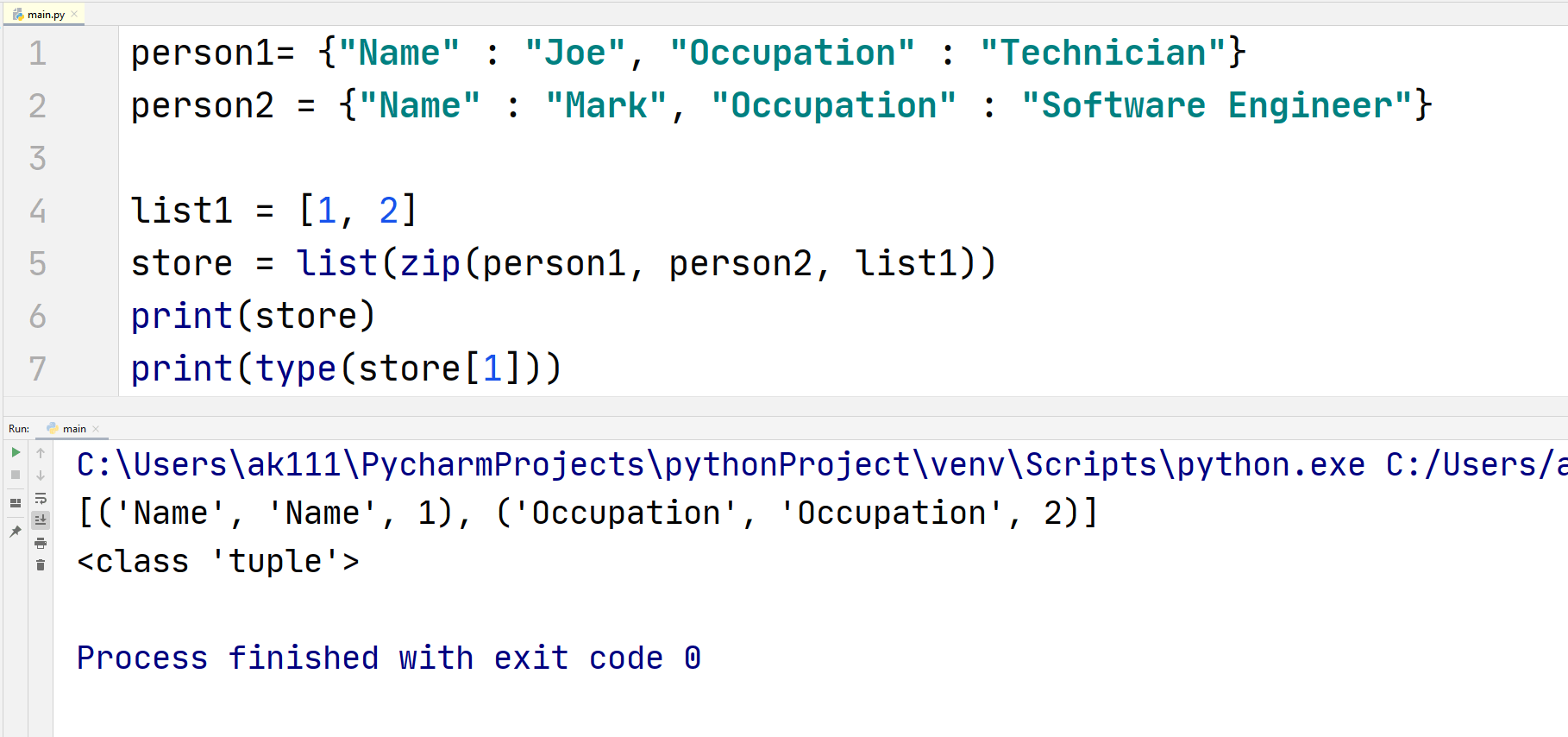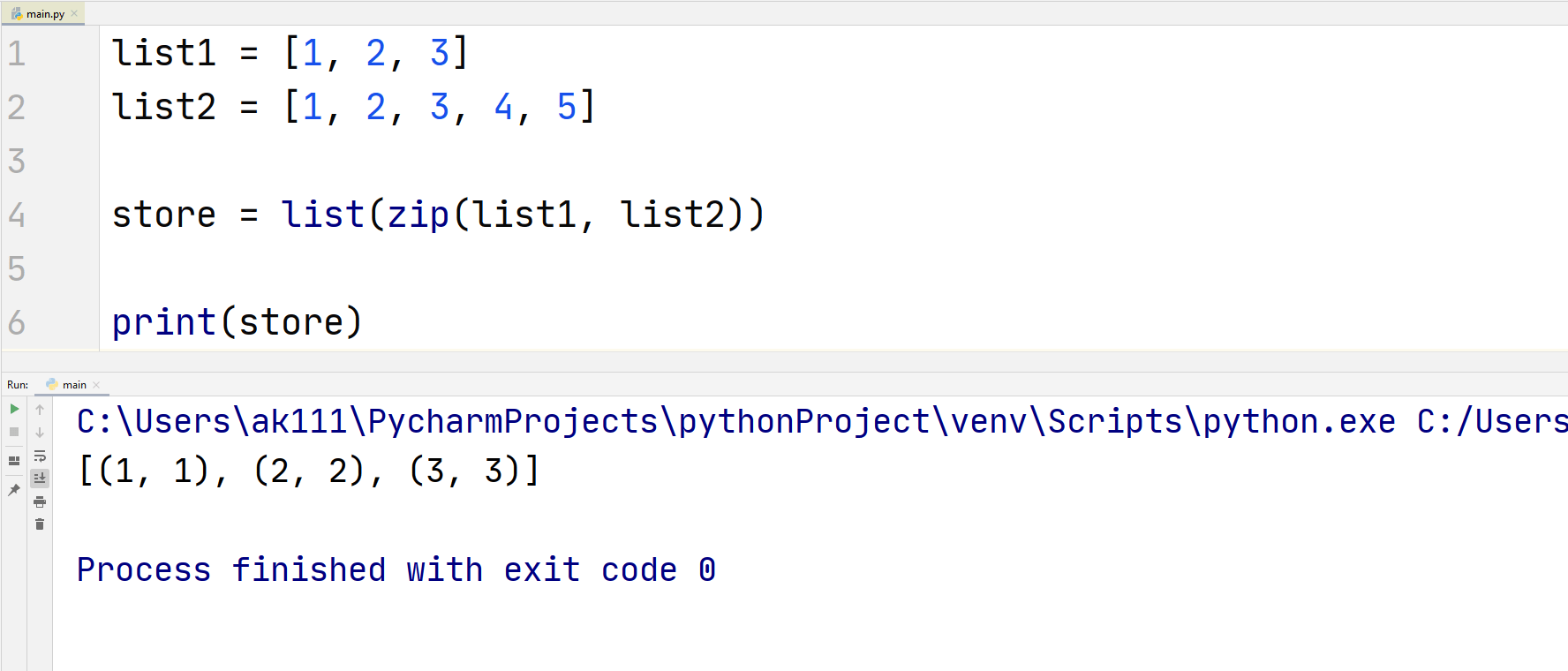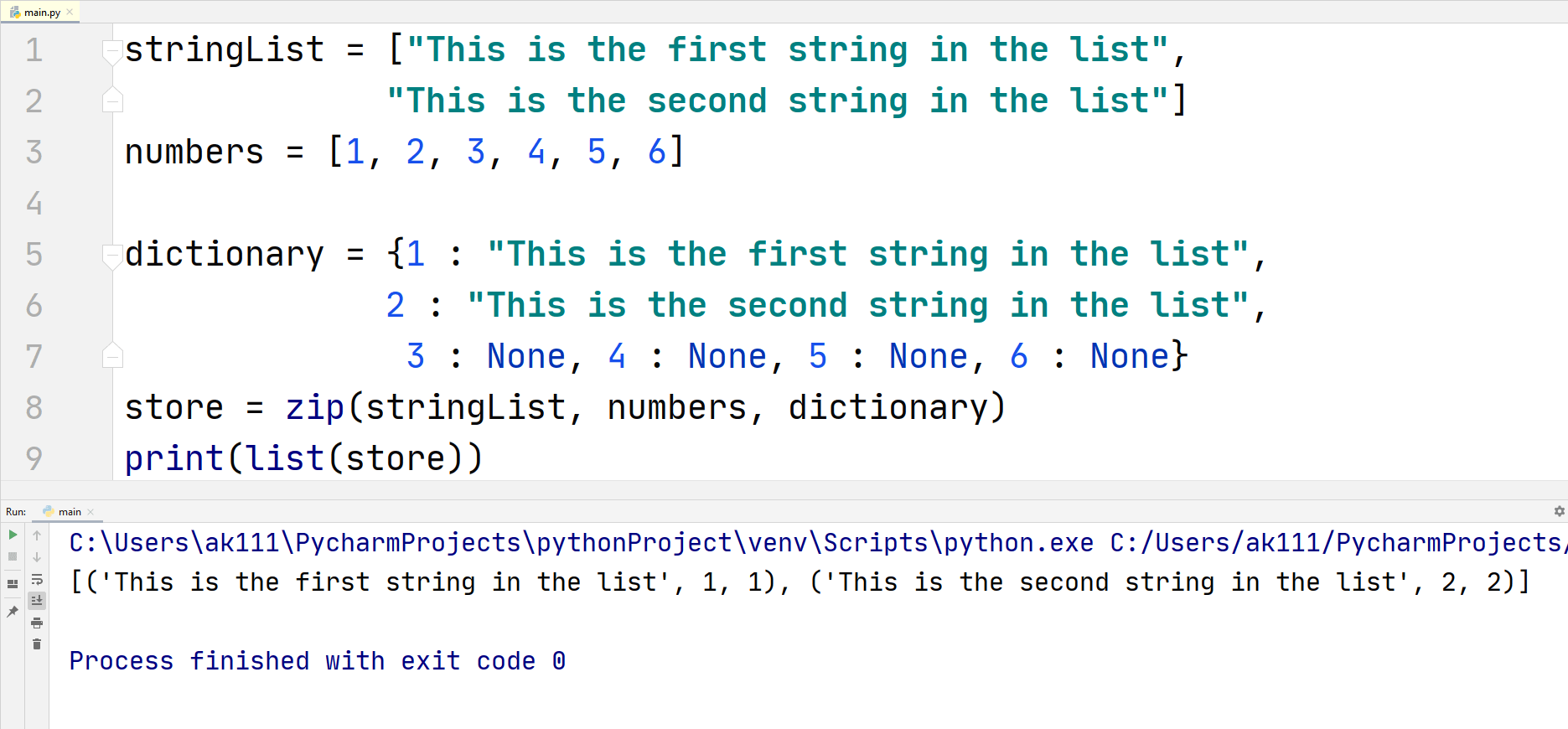# Python Zip

## Python Zip

What is the zip() function? No, it is not used to make a zip file. The Python zip() function is used to contain multiple iterables together and returns them as another iterable.

It is an in-built module in Python, which means that you will not have to import any module to use this function.

This in-built Python function can take any number of arguments, but the arguments that are passed must be iterables. For example, if you pass an integer in the function, then Python would show you a TypeError.

Syntax
zip(*iterables)

## Using zip()

This is how you can use the zip() function in Python to contain multiple iterators together.

## ExampleThis is generally used to store related information, it can make the values more accessible and relevant.

Code Explanation:

We pass our variables (two dictionary variables and a list) that we created into the zip function, the zip function binds them together and returns tuple values enclosed in whatever iterable type we specify!

Furthermore, we confirm that the returned object is really a Tuple by printing the type of the store variable using the type() function.

## Specify Type

If you want the zip() function to return something else other than a list, you can do that too. A zip object can be converted to a dictionary too, let’s have an example:

## ExampleYou can see in the output that the object that got returned is of type “list”. You can use this to convert the zip object in any iterable except a string. But what happens when you try to convert the zip object into a string, we shall find out:

## ExampleIt returns an address where our zip object variable is stored instead of giving us a string value of the variable.

## zip() Working

The zip() function does not work as simply as you expect it to be. Below is how it will work under some conditions:

• If you do not pass any iterable as parameters in the zip() function then the function will only return an empty Tuple with length = 0.

If only a single iterable is passed in the parameter, then the zip() function will return an iterable containing a number of tuples equal to the number of elements in the iterable.

## Example• You can see that the list we get in the output has two tuples inside it. We confirm this by checking the size of the list.

If you pass multiple iterables in the function, the number of tuple that it will return would be equal to the length of the shortest iterable passed.

For example, if you passed two iterables in the zip() function, one having 3 elements and another one with 5 elements then, the output will only have 3 elements with each element having one-one iterators from both iterators.

Consider this as an example for better understanding:

## ExampleAs you can see we only get three tuples in the list, this is because the zip() function exhausted the smallest iterable.
This means that you should have an equal number of values in each iterable to include all of the iterable values.

Tips
If you want to use the zip() function correctly, you will need to understand these points better.

## Zipping a Dictionary

The Python’s zip() function works differently for a dictionary, when you print the zip variable, it will not contain the whole dictionary.

## ExampleThis program only returned the keys inside the dictionary. These keys can be used to access the values inside the dictionary. Also, note that we used the None keyword to assign empty values to the keys.

### Unzip using zip()

You can get all the values that you zipped in the original form back from the zip() function like this.

## ExampleWe got the original form back like we wanted. The comma separated values a and b specified that whatever parameters are in the zip function should be equal to them. Then, we simply printed out the values of a and b.